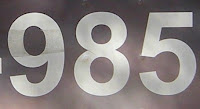## Thursday, February 11, 2010

### 985

985 = 5 x 197.

985 has two representations as a sum of two squares: 985 = 122 + 292 = 162 + 272.

985 is the hypotenuse of two primitive Pythagorean triples: 9852 = 4732 + 8642 = 6962 + 6972. It is the fourth Pythagorean triple where two sides are consecutive integers.

985 is the ninth Pell number.985 is the telephone area code for southeastern Louisiana.